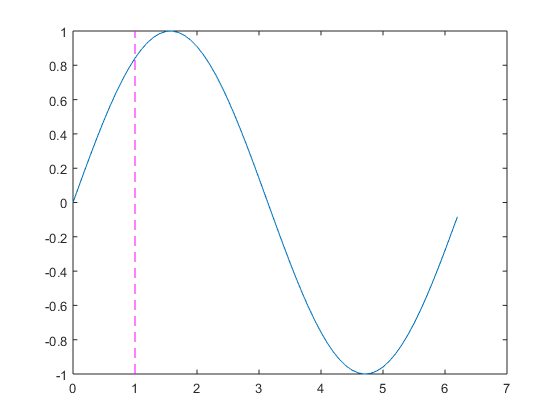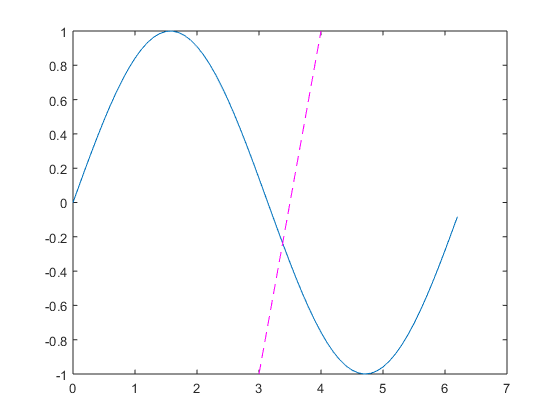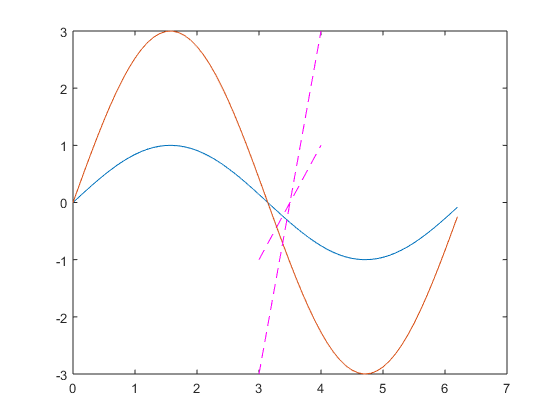# matlab绘制平行于坐标轴的直线

[plain] view plain
1. x=0:0.1:2*pi;   %x的取值生成
2. y=sin(x);   %y=sin(x)函数
3. plot(x,y);  %绘制图形
4. ylim=get(gca,'Ylim'); % 获取当前图形的纵轴的范围
5. hold on
6. plot([1,1],ylim,'m--'); % 绘制x=1的直线
7. %[1,1]中，第一个1表示绘制直线的穿过x轴点，第2个1表示直线穿过y轴的另个点，由此两点确定想要绘制直线的方向
8. %ylim 用于绘制y轴的取值范围
9. %m-- 用于描述线型，粉色，虚线[plain] view plain
1. x=0:0.1:2*pi;   %x的取值生成
2. y=sin(x);   %y=sin(x)函数
3. plot(x,y);  %绘制图形
4. ylim=get(gca,'Ylim'); % 获取当前图形的纵轴的范围
5. hold on
6. plot([3,4],ylim,'m--'); % 绘制直线
7. %ylim 用于绘制y轴的取值范围
8. %m-- 用于描述线型，粉色，虚线[plain] view plain
1. x=0:0.1:2*pi;   %x的取值生成
2. y=3*sin(x);   %y=sin(x)函数
3. plot(x,y);  %绘制图形
4. ylim=get(gca,'Ylim'); % 获取当前图形的纵轴的范围
5. hold on
6. plot([3,4],ylim,'m--'); % 绘制x=1的直线
7. %ylim 用于绘制y轴的取值范围
8. %m-- 用于描述线型，粉色，虚线[plain] view plain
1. ylim=get(gca,'Ylim'); % 获取当前图形的纵轴的范围